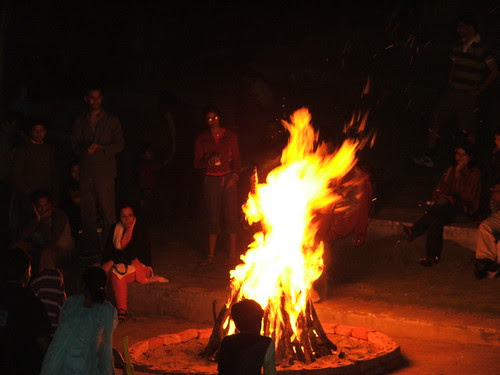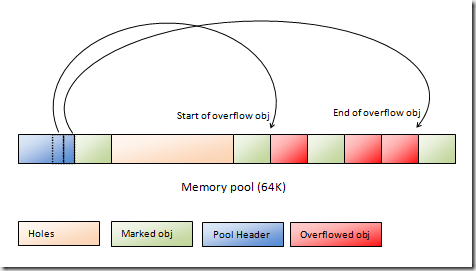## Friday, February 20, 2009

### Back To Basics: Handling overflow in mark stageThis post is Part 7 in the series of posts on Garbage Collection (GC). Please see the index here.

Let’s first recap the basic algorithm of the mark-sweep garbage collection. In C like pseudo code the algorithm looks like

`void GC(){    HaltAllThreads();    ObjectCollection roots = GetRoots();    for(int i = 0; i < roots.Count(); ++i)        Mark(roots[i]);    Sweep();}void Mark(Object* pObj){    if (!Marked(pObj)) // Marked returns the marked flag from object header    {        MarkBit(pObj); // Marks the flag in obj header        // Get list of references that the current object has        // and recursively mark them as well        ObjectCollection children = pObj->GetChildren();        for(int i = 0; i < children.Count(); ++i)        {            Mark(children[i]); // recursively call mark        }    }}`

This algorithm is by nature recursive where in the marking phase we recursively mark every object and it’s children. However, recursion is not optimum in terms of both time and space usage. Each method call comes with additional overhead which is particularly more bothersome because GC is anyway run when the system is low on resource.

Controlling the depth of call stack in recursive algorithm is also hard. In the algorithm shown above the depth of the stack is equal to the length of the longest reference chain.

This is worked around by using general recursion to iteration conversion methods like using explicit marking stacks as show below

`Stack g_MarkStack(MAX_SIZE);void Init(){    g_MarkStack.RemoveAll();}void GC(){    HaltAllThreads();    Init();    ObjectCollection roots = GetRoots();    for(int i = 0; i < roots.Count(); ++i)        g_MarkStack.Push(roots[i]);    Mark();    Sweep();}void Mark(){    while(!g_MarkStack.Empty())    {        Object *pObj = g_MarkStack.Pop();        if(!Marked(pObj))        {            MarkBit(pObj);            ObjectCollection children = pObj->GetChildren();            for(int i = 0; i < children.Count(); ++i)            {                g_MarkStack.Push(children[i]);            }        }    }}`

Using explicit marking stack we have removed the need for recursive algorithm. Now this is more deterministic and it is easy to figure out when the heap graph is too deep and the stack actually overflows (at the point of push we can check for g_MarkStack.IsFull())

### Handling overflow

Explicit stack makes detecting and handling of stack overflow easier. It doesn’t however, remove the issue. The first cut attempt is to reduce the number of items being pushed into the stack. Objects are pushed onto the stack so that they behave as branch points so that once a child has been marked we can come back to it’s parent and branch off and mark it’s other children. So simple fixes like only pushing in case an object has two or more children does help in reducing the overflows

There are other ways to work around this problem like using alternate tree marking algorithm like Schorr-Waite graph marking algorithm

However, whatever method we try there will be large objects trees where the stack will run out of space and that needs to be handled.

.NET Compact framework uses a two level fall back scheme as explained below.

Using overflow stack

The basic mark stack used is a grow-able stack buffer which can grow to max 4KB. In case this overflows another small fixed sized stack is used which is called the overflow-stack. Objects where the overflow happens while marking are pushed on to this overflow stack. The children of these are not traversed.

This means a part of the tree is left out from marking and growth of the mark stark is kind of contained. The mark buffer unwinds and marking is started back on it. In the next pass marking is restarted considering the objects on the overflow-stack as roots. This is done iteratively. The idea here is that with each such pass, more and more of the tree is marked and hence the marking finally converges.

Overflow-arena

For really deep trees even the overflow-stack can overflow. To handle this the following steps are taken

1. .NETCF uses pooled memory where 64K sized memory pools are used and objects are sub-allocated from them. The memory pool where the overflowed object resides is located. Each of these pools contain two pointers which point to the first and the last objects in the pool that overflowed. These two pointers are updated to point to the currently overflowed object.
2. The header of the object where the overflow happened is marked with a special bit flag OBJFLAG_MARK_OVERFLOW.
3. At this point the object pool looks something like4. A global linklist of all such pools which has overflowed objects is also preserved
5. Once objects in mark-stack and overflow-stack is marked another pass is started which does the following
foreach Pool in Overflow-arena